##### The Japanese Maple specialist
Direct order Contact Help / Services Newsletter# Shelf to displaySearch

Japanese MaplesYoung trees Acer seeds and more Bonsai soil and fertiliserVarieties introduced into EuropeHostasWind bells and chimesSaxifragesSpecial offer

Packing and shipping charges

# Crataegifolium veitchi

Crataegifolium veitchi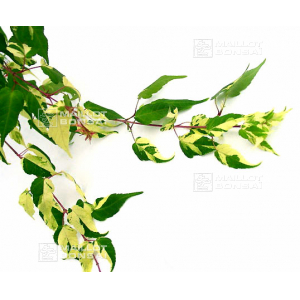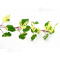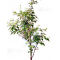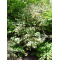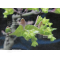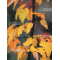ref. : 11906

Choose the right size
Pot size

This item is temporarily unavailable

###### Description

A low-growing maple with variegated leaves of creamy white, green and pink. in spring and then in summer on new shoots. Orange fall color. The young branches are red and the trunk as it ages becomes gray-white veined. It is classified as a snakeskin maple. Inflorescences appear drooping in clusters.
Native to the humid mountains of Japan. Discovered in 1879.

#crataegifolium 3.4 #japanese 2.8 #veitchi 2.7 #maples 2.6 #maple 2.5 #white 2.5 #inflorescences 2.4 #discovered 2 #variegated 2 #classified 2

###### Technical description
 Species Japanese Maples same species items Variety Crataegifolium veitchi Leaf colour Variegated leaves same colour items Leaf shape Others same shaped items Adult height Less than 2 meters same height items Shape Natural same shape items Exposure Sun same exposure items

Formule
(( ROUND((CHAR_LENGTH(b.article_nom)-CHAR_LENGTH(REPLACE(b.article_nom, 'crataegifolium', '')))/LENGTH('crataegifolium')) + ROUND((CHAR_LENGTH(b.article_description)-CHAR_LENGTH(REPLACE(b.article_description, 'crataegifolium', '')))/LENGTH('crataegifolium')) ) * 3.4) + (( ROUND((CHAR_LENGTH(b.article_nom)-CHAR_LENGTH(REPLACE(b.article_nom, 'veitchi', '')))/LENGTH('veitchi')) + ROUND((CHAR_LENGTH(b.article_description)-CHAR_LENGTH(REPLACE(b.article_description, 'veitchi', '')))/LENGTH('veitchi')) ) * 2.7) + (( ROUND((CHAR_LENGTH(b.article_nom)-CHAR_LENGTH(REPLACE(b.article_nom, 'white', '')))/LENGTH('white')) + ROUND((CHAR_LENGTH(b.article_description)-CHAR_LENGTH(REPLACE(b.article_description, 'white', '')))/LENGTH('white')) ) * 2.5) + (( ROUND((CHAR_LENGTH(b.article_nom)-CHAR_LENGTH(REPLACE(b.article_nom, 'maple', '')))/LENGTH('maple')) + ROUND((CHAR_LENGTH(b.article_description)-CHAR_LENGTH(REPLACE(b.article_description, 'maple', '')))/LENGTH('maple')) ) * 2.5) + (( ROUND((CHAR_LENGTH(b.article_nom)-CHAR_LENGTH(REPLACE(b.article_nom, 'inflorescences', '')))/LENGTH('inflorescences')) + ROUND((CHAR_LENGTH(b.article_description)-CHAR_LENGTH(REPLACE(b.article_description, 'inflorescences', '')))/LENGTH('inflorescences')) ) * 2.4) + (( ROUND((CHAR_LENGTH(b.article_nom)-CHAR_LENGTH(REPLACE(b.article_nom, 'discovered', '')))/LENGTH('discovered')) + ROUND((CHAR_LENGTH(b.article_description)-CHAR_LENGTH(REPLACE(b.article_description, 'discovered', '')))/LENGTH('discovered')) ) * 2) + (( ROUND((CHAR_LENGTH(b.article_nom)-CHAR_LENGTH(REPLACE(b.article_nom, 'variegated', '')))/LENGTH('variegated')) + ROUND((CHAR_LENGTH(b.article_description)-CHAR_LENGTH(REPLACE(b.article_description, 'variegated', '')))/LENGTH('variegated')) ) * 2) + (( ROUND((CHAR_LENGTH(b.article_nom)-CHAR_LENGTH(REPLACE(b.article_nom, 'classified', '')))/LENGTH('classified')) + ROUND((CHAR_LENGTH(b.article_description)-CHAR_LENGTH(REPLACE(b.article_description, 'classified', '')))/LENGTH('classified')) ) * 2) + (( ROUND((CHAR_LENGTH(b.article_nom)-CHAR_LENGTH(REPLACE(b.article_nom, 'snakeskin', '')))/LENGTH('snakeskin')) + ROUND((CHAR_LENGTH(b.article_description)-CHAR_LENGTH(REPLACE(b.article_description, 'snakeskin', '')))/LENGTH('snakeskin')) ) * 1.9) + (( ROUND((CHAR_LENGTH(b.article_nom)-CHAR_LENGTH(REPLACE(b.article_nom, 'mountains', '')))/LENGTH('mountains')) + ROUND((CHAR_LENGTH(b.article_description)-CHAR_LENGTH(REPLACE(b.article_description, 'mountains', '')))/LENGTH('mountains')) ) * 1.9)

## Secure payment## Delivery

Our logistic partners :04 74 55 23 48
Pépinière MAILLOT-BONSAÏ
Le Bois Frazy
01990 RELEVANT - FRANCE
on appointment